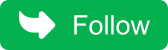## Wednesday, May 4, 2022

### Question 31 : Check if Array Elements are Consecutive

Given an array, we need to check if array contains consecutive elements.
For example :
``````Input: array[] = {5, 3, 4, 1, 2}
Output: true
As array contains consecutive elements from 1 to 5
Input: array[] = {47, 43, 45, 44, 46}
Output: true
As array contains consecutive elements from 43 to 47
Input: array[] = {6, 7, 5, 6}
Output: false
As array does not contain consecutive elements.``````

## Solution

A simple solution will be to sort the array and check if elements are consecutive just by iterative over the array but the time complexity of this solution will be o(n^logn).

Can we do better?
Yes, we can do better

• Find minimum and maximum element in the array.
• Check if max-min+1==n, if elements are consecutive then this condition should meet.
• Create a visited boolean array.
• Iterate over the array and check
• visited[arr[i]-min] is true, then return false as elements are repeated.
• mark the element visited.

### Program to check if Array Elements are Consecutive

``````public class ArrayConsecutiveElementMain{

/* Method return minimum value*/
private int getMinimum(int arr[], int n)
{
int min = arr;
for (int i = 1; i < n; i++)
if (arr[i] < min)
min = arr[i];
return min;
}

/* Method return maximum value*/
private int getMaximum(int arr[], int n)
{
int max = arr;
for (int i = 1; i < n; i++)
if (arr[i] > max)
max = arr[i];
return max;
}

/* This method checks if array elements are consecutive */
public boolean checkArrayContainsConsecutiveElements(int arr[], int n)
{
if ( n <  1 )
return false;

int min = getMinimum(arr, n);
int max = getMaximum(arr, n);

if (max - min  + 1 == n)
{
boolean[] visited=new boolean[arr.length];
for (int i = 0; i < n; i++)
{
if ( visited[arr[i] - min] != false )
return false;

visited[arr[i] - min] = true;
}

return true;
}
return false;
}

public static void main(String args[])
{
ArrayConsecutiveElementMain acem=new ArrayConsecutiveElementMain();
int arr[]= {47, 43, 45, 44, 46};

if(acem.checkArrayContainsConsecutiveElements(arr, arr.length))
System.out.println(" Array elements are consecutive ");
else
System.out.println(" Array elements are not consecutive ");
return;
}
}
``````

When you run the above program, you will get the below output:

``Array elements are consecutive``

The time complexity of this solution is o(n).

Don't miss the next article!
Be the first to be notified when a new article or Kubernetes experiment is published.